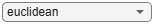Documentation

## Fuzzy C-Means Clustering

This example shows how to perform fuzzy c-means clustering on 2-dimensional data. For an example that clusters higher-dimensional data, see Fuzzy C-Means Clustering for Iris Data.

Fuzzy c-means (FCM) is a data clustering technique in which a data set is grouped into N clusters with every data point in the dataset belonging to every cluster to a certain degree. For example, a data point that lies close to the center of a cluster will have a high degree of membership in that cluster, and another data point that lies far away from the center of a cluster will have a low degree of membership to that cluster.

The `fcm` function performs FCM clustering. It starts with a random initial guess for the cluster centers; that is the mean location of each cluster. Next, `fcm` assigns every data point a random membership grade for each cluster. By iteratively updating the cluster centers and the membership grades for each data point, `fcm` moves the cluster centers to the correct location within a data set and, for each data point, finds the degree of membership in each cluster. This iteration minimizes an objective function that represents the distance from any given data point to a cluster center weighted by the membership of that data point in the cluster.

Load the five sample data sets, and select a data set to cluster. These data sets have different numbers of clusters and data distributions.

```load fcmdata dataset =fcmdata3;```

### Specify FCM Settings

Configure the clustering algorithm settings. For more information on these settings, see `fcm`. To obtain accurate clustering results for each data set, try different clustering options.

Specify the number of clusters to compute, which must be greater than `1`.

`N =4;`

Specify the exponent the fuzzy partition matrix, which controls the degree of fuzzy overlap between clusters. This value must be greater than `1`, with smaller values creating more crisp cluster boundaries. For more information, see Adjust Fuzzy Overlap in Fuzzy C-Means Clustering.

`exponent =2;`

Specify the maximum number of optimization iterations.

`maxIterations =100;`

Specify the minimum improvement in the objective function between successive iterations. When the objective function improves by a value below this threshold, the optimization stops. A smaller value produces more accurate clustering results, but the clustering can take longer to converge.

`minImprovement =0.00001;`

Specify whether to display the objective function value after each iteration.

`displayObjectiveFunction =false;`

Create an option vector for the `fcm` function using these settings.

`options = [exponent maxIterations minImprovement displayObjectiveFunction];`

### Cluster Data

Cluster the data into `N` clusters.

`[C,U] = fcm(dataset,N,options);`

`C` contains the computed centers for each cluster. `U` contains the computed fuzzy partition matrix, which indicates the degree of membership of each data point within each cluster.

Classify each data point into the cluster for which it has the highest degree of membership.

```maxU = max(U); index = cell(N,1); for i=1:N index{i} = find(U(i,:) == maxU); end```

### Plot Clustering Results

Plot the clustering results.

```figure hold on for i=1:N plot(dataset(index{i},1),dataset(index{i},2),'o') plot(C(i,1),C(i,2),'xk','MarkerSize',15,'LineWidth',3) end hold off```The data points in each cluster are shown in a different colors. The center for each cluster is shown as a black `X`.

### Plot Data Point Membership Values

Select a cluster for which to plot a membership function surface.

`cluster =2;`

Obtain the membership function for the selected cluster by fitting a surface to the cluster membership values for all data points. For more information on interpolating scattered 3-D data, see `griddata`.

```[X,Y] = meshgrid(0:0.05:1, 0:0.05:1); Z = griddata(dataset(:,1),dataset(:,2),U(cluster,:),X,Y); surf(X,Y,Z)```When you decrease the `exponent` value, the transition from maximum full cluster membership to zero cluster membership becomes more steep; that is, the cluster boundary becomes more crisp.